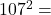## alculating Displacement under Constant Acceleration Use the information from the graph to answer the question What is the

Question

alculating Displacement under Constant Acceleration
Use the information from the graph to answer the
question
What is the total displacement of the object?
m
Velocity vs. Time
40
30
Velocity (m/s)
20
10
0
0
5
10
15
20
25
Time (s)
Intro
Done
ODO
ODDO
tivity

in progress 0
6 months 2021-07-13T19:38:52+00:00 1 Answers 22 views 0